Thank you for visiting nature.com. You are using a browser version with limited support for CSS. To obtain the best experience, we recommend you use a more up to date browser (or turn off compatibility mode in Internet Explorer). In the meantime, to ensure continued support, we are displaying the site without styles and JavaScript.

# The Chaos Within Sudoku

## Abstract

The mathematical structure of Sudoku puzzles is akin to hard constraint satisfaction problems lying at the basis of many applications, including protein folding and the ground-state problem of glassy spin systems. Via an exact mapping of Sudoku into a deterministic, continuous-time dynamical system, here we show that the difficulty of Sudoku translates into transient chaotic behavior exhibited by this system. We also show that the escape rate κ, an invariant of transient chaos, provides a scalar measure of the puzzle's hardness that correlates well with human difficulty ratings. Accordingly, η = −log10 κ can be used to define a “Richter”-type scale for puzzle hardness, with easy puzzles having 0 < η ≤ 1, medium ones 1 < η ≤ 2, hard with 2 < η ≤ 3 and ultra-hard with η > 3. To our best knowledge, there are no known puzzles with η > 4.

## Introduction

In Sudoku, considered as one of the world's most popular puzzles1, we have to fill in the cells of a 9 × 9 grid with integers 1 to 9 such that in all rows, all columns and in nine 3 × 3 blocks every digit appears exactly once, while respecting a set of previously given digits in some of the cells (the so-called clues). Sudoku is an exact cover type constraint satisfaction problem2 and it is one of Karp's 21 NP-complete problems3, when generalized to N × N grids4. NP-complete problems are “intractable” (unless P = NP)2,5 in the sense that all known algorithms that compute solutions to them do so in exponential worst-case time (in the number of variables N); in spite of the fact that if given a candidate solution, it takes only polynomial time to check its correctness.

The intractability of NP-complete problems has important consequences, ranging from public-key cryptography to statistical mechanics. In the latter case, for the ground-state problem of Ising spin glasses (±1 spins), one needs to find the lowest energy configuration among all the 2N possible spin configurations, where N is the number of spins. Additionally, to describe the statistical behavior of such Ising spin models, one has to compute the partition function, which is a sum over all the 2N configurations. Barahona6, then Istrail7 have shown that for non-planar crystalline lattices, the ground-state problem and computing the partition function are NP-complete7. Since there is little hope in providing polynomial time algorithms for NP-complete problems, the focus shifted towards understanding the nature of the complexity forbidding fast solutions to these problems. There has been considerable work in this direction, especially for the boolean satisfiability problem SAT (or k-SAT), which is NP-complete for k ≥ 3. Completeness means that all problems in NP (hence Sudoku as well), can be translated in polynomial time and formulated as a k-SAT problem, as shown for the first time by Cook and Levin2. Namely, any problem in NP can be solved via a small number of calls to a k-SAT solver and a polynomial number of steps (in the size of the input) outside the subroutine invoking the k-SAT solver.

In k-SAT we are given N boolean variables to which we need to assign 1s or 0s (TRUE or FALSE) such that a given set of clauses in conjunctive normal form, each containing k or fewer literals (literal: a boolean variable or its negation) are all satisfied, i.e., evaluate to TRUE. Just as for the spin glass model, here we also have exponentially many (2N) configurations or assignments to search.

In the following we treat algorithms as dynamical systems. An algorithm is a finite set of instructions acting in some state space, applied iteratively from an initial state until an end state is reached. For example, the simplest algorithm for the Ising model ground state problem, or the 3-SAT problem would be exhaustively testing potentially all the 2N configurations, which quickly becomes forbidding with increasing N. To improve performance, algorithms have become more sophisticated by exploiting the structure of the problem (of the state space). Accordingly, now 3-SAT can be solved by a deterministic algorithm with an upper bound of O(1.473N) steps8. Here we will only deal with deterministic algorithms that is, once an initial state is given, the “trajectory” of the dynamical system is uniquely determined. Thus, we expect that the dynamics of those algorithms that exploit the structure of hard problems will reflect the complexity inherent in the problem itself. Complex behavior by deterministic dynamical systems is coined chaos in the literature9,10,11,12,13 and thus the behavior of algorithms for hard problems is expected to appear highly irregular or chaotic14.

Although the theory of nonlinear dynamical systems and chaos is well-established, it has not yet been exploited in the context of optimization algorithms. One of the difficulties lies with the fact that most optimization algorithms are discrete and not easily cast in forms amenable to chaos theory methods. Recently, however, we have provided15 a deterministic continuous-time solver for the boolean satisfiability problem k-SAT using coupled ordinary differential equations (ODE) with a one-to-one correspondence between the k-SAT solution clusters and the attractors of the corresponding system of ODEs. This continuous-time dynamical system (CTDS) is in a form naturally suited for chaos theory methods and thus it allows us to study the relationship between optimization hardness and chaotic behavior. Here we will focus only on solvable (satisfiable) instances and thus the observed chaotic behavior will necessarily be transient11,12,13,16,17. We need to emphasize, however, that the dynamical properties characterize both the problem and the algorithm itself. For this reason, one compares the dynamical properties across problems of varying hardness using the same algorithm. Nevertheless, since there are problem instances that are hard for all known algorithms, the appearance of transient chaos with long lifetime should be a universal feature of hard problems. It is also important to observe that transient chaos is not an N → ∞ asymptotic behavior (where N is the size of the problem in terms of input or number of variables), but it appears for finite N and thus measures of chaos can be used to characterize and categorize the hardness of individual instances of finite problems. To illustrate this, here we first map the popular 9 × 9 (hence finite) version of Sudoku into k-SAT, then we solve it using our deterministic continuous-time solver15. By analyzing the behavior of the corresponding trajectories of the CTDS we show the appearance of transient chaos when increasing the hardness of the Sudoku problems and show that the level of hardness (taken from human ratings of the puzzles) correlates well with a chaotic invariant, namely the lifetime of chaos estimated as κ−1, where κ is called the escape rate11. We conclude with a discussion on algorithmic performance, dynamical properties and problem complexity.

## Results

### Sudoku as k-SAT

Because our continuous-time dynamical system15 was designed to solve k-SAT formulae in conjunctive normal form (CNF), we first briefly describe how Sudoku can be interpreted as a +1-in-9-SAT formula and then how it is transformed into the standard CNF form. Further details are shown in the Methods section.

In a Sudoku puzzle we are given a square grid with 9 × 9 = 81 cells, each to be filled with one of nine symbols (digits), i, j = 1, …, 9 (with the upper-left corner of the puzzle corresponding to i = 1, j = 1). When the puzzle is completed, each of the columns, rows and 3 × 3 sub-grids (blocks partitioned by bold lines, Fig. 1a) must contain all the 9 symbols. Equivalently, all 9 symbols must appear once and only once in each row, column and 3 × 3 sub-grid.

To formulate Sudoku as a constraint satisfaction problem (CSP) using boolean variables, we associate to each symbol (digit) an ordered set of 9 boolean variables (TRUE = “1”, FALSE = “0”). The digit Dij in cell (i, j) will be represented as the ordered set () with, a = 1, …, 9, such that always one and only one of them is 1 (TRUE). In particular, Dij = a is set to be equivalent to, where δa,b is the Kronecker delta function. This way we have in total 9 × 9 × 9 = 729 boolean variables, which we can picture as being placed on a 3D grid (Fig. 1b), with a corresponding to the grid index along the vertical direction and hence a is the digit that is filling the corresponding (i, j) cell in the original puzzle. The corresponding horizontal 9 × 9 2D layer at height a will be denoted by La. Introducing the notion of such (horizontal) layers makes it easier to express the constraints of the Sudoku rules on its representation by 0-s and 1-s as described below. For example in the puzzle shown in Fig. 1a, we have D1,9 = 4. In the given vertical column the variable in the ath cell (that is in layer La) is(shown as the boolean variable 1 filling the cell next to the digit 4 shown in red, in Fig 1b). This setup allows us to encode the Sudoku constraints in a simple manner. They come from: 1) uniqueness of the digits in all the (i, j) Sudoku cells, 2) a digit must occur once and only once in each row, column and in each of the nine 3 × 3 subgrids and 3) obeying the clues. Constraint type 1) was already expressed above, namely that for every cell (i, j), in the set () one and only one variable is TRUE, all others must be FALSE. Type 2) constraints are similar, e.g., in row i and layer La the set () must contain one and only one TRUE variable, all others must be false and this must hold for all rows and layers, etc. Observe that all constraints are in the form of a set of 9 boolean variables of which we demand that one and only one of them be TRUE, all others FALSE. When this is satisfied, we say that the constraint itself (or “clause”) is satisfied, or TRUE. Such CSPs are called +1-in-k-SAT and they are part of so-called “locked occupation problems”, which is a class of exceptionally hard CSPs18,19. Type 3) constraints are generated by the clues (or givens) which are symbols already filled in some of the cells and their number and positioning determines the difficulty of the puzzle. They are also set in a way to guarantee a unique solution to the whole puzzle. If there are given d clues, then this implies setting d boolean variables to TRUE, which means eliminating exactly 4d constraints of type 1) and 2) (one vertical or uniqueness constraint, one row, one column and one 3 × 3 subgrid constraint). Thus, Sudoku is a +1-in-9-SAT type CSP with N boolean variables and 324 − 4d constraints of +1-in-k-SAT type (k ≤ 9). N is a complicated function of the positioning of the clues. The example in Fig. 1a has d = 22 clues with N = 232 unknown boolean variables. In layer L4 as illustrated in Fig. 1c there are 28 unknown boolean variables (white cells). These 28 variables appear in a total of 17 constraints of +1-in-k-SAT type. More precisely there is one +1-in-2-SAT, six +1-in-4-SAT, four +1-in-5-SAT and six +1-in-6-SAT type of constraints related to L4. The other layers generate the remaining 324 − 4d − 17 = 219 of +1-in-k-SAT type constraints (with k ≤ 9).

Since our continuous-time SAT solver has been designed to solve boolean satisfiability problems in conjunctive normal form (CNF), we need to bring the +1-in-k-SAT type problems above into this form and thus formulate it as a k-SAT CNF problem. The CNF is a conjunction (AND, denoted by ) of clauses each clause expressed as the disjunction (OR, denoted by ) of literals. For k-SAT in CNF there are N boolean variables xi = {0, 1} and an instance is given as a propositional formula, which is the conjunction of M clauses Cm: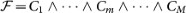, with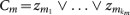, kmk and zj is a literal, that is(is the negation of xj). According to a well known theorem of propositional calculus, all boolean propositions can be formulated in CNF using De Morgan's laws and the distributive law and thus any +1-in-k-SAT type constraint as well. The Methods section describes how to translate +1-in-k-SAT type constraints into CNF.

Once the transformation to CNF is completed we are left with N variables and M clauses of the type described above, called CNF clauses from here on. In our case the number of variables appearing in a CNF constraint has the property 1 ≤ km ≤ 9. The parameters N, M anddepend on the clues that are difficult to express analytically, but easy to determine computationally. The puzzle from Fig. 1a is ultimately formulated as a CNF SAT problem with N = 232 variables and M = 1718 CNF clauses. An often used parameter of a satisfiability problem is the number of CNF constraints per variable, or constraint density, α = M/N, also used as a typical hardness indicator, however, as we show below, this is not an accurate measure of hardness.

### The continuous-time deterministic k-SAT solver

In Ref  a continuous-time deterministic solver was introduced to solve k-SAT problems in conjunctive normal form. The set of clauses specifying the constraints are translated into an M × N matrix: C = {cmi} with cmi = 1 if the variable xi is present in clause m in direct (non-negated) form, namely, cmi = −1 ifand cmi = 0 if xi andare both absent from Cm. To every variable xi one associates a continuous spin variablesuch that when si = ±1 then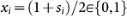and to every clause Cm one associates the function: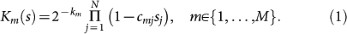We havefor all. It is easy to check that Km = 0 only for thosevalues for which the corresponding xi-s satisfy clause Cm (otherwise we always have Km > 0). That is, Km plays the role of an energy function for clause Cm and its ground state value of Km = 0 is reached if Cm is TRUE and only then. We also need the quantities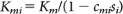that is, with the i-th term missing from the product in (1). Clearly,. The continuous time dynamical system introduced in15 is defined via the set of (N + M) ordinary differential equations (ODEs):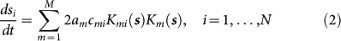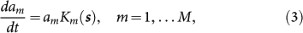with the only requirements that, i and am(0) > 0, m. The latter implies from (3) that am(t) > 0, m, t. It was shown in Ref  that system (2–3) always finds the solutions to k-SAT problems (encoded via the C matrix), when they exist, from almost all initial conditions (the exception being a set of Lebesgue measure zero). Here we give an intuitive picture for why that is the case. Due to (3) the auxiliary variables am grow exponentially at rate Km. That is, the further is Km from its ground-state value of 0, the faster am grows (in that instant). Moreover, the longer has Km been away from zero, the larger is am, as seen from the formal solution to (3):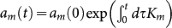. Equation (2) can equivalently be written as a gradient descent on an energy landscape V(s, a), that is ds/dt = − sV, where s is the gradient operator in the spin variables and. Clearly, V ≥ 0 t and V = 0 if and only if s is a k-SAT solution, i.e., satisfies all the clauses (Km(s) = 0, m).

From the behavior of the am variables discussed above it also follows that the least satisfied constraints will dominate V (terms with the largest am-s). Without restricting generality, let theterm be the most dominant at t. Then keeping only the dominant term on the rhs of (2) for those i for which c1i ≠ 0 we get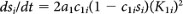or, equivalently: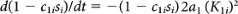. This shows that the term (1 − c1isi) is driven exponentially fast towards zero, that is towards satisfying K1 (and all the other constraints containing this term). As K1 decreases, some other constraint becomes dominant and thus, in a continuous fashion, all constraints are driven towards satisfiability. The exponential growth guarantees that the trajectory is always pulled out of any potential well. When the problem is unsatisfiable, the system generates a chaotic dynamics in [−1, 1]N, indefinitely. For more details about the properties of the continuous-time dynamical system (CTDS) (2–3) see Ref. .

### Puzzle hardness as transient chaotic dynamics

Since Sudoku puzzles always have a solution, the corresponding boolean SAT CNF formulation also has a solution and system (2-3) will always find it. The nature of the dynamics, however, will depend on the hardness of the puzzle as we describe next.

In Fig.2a we show an easy puzzle with 34 clues (black numbers)20. After transforming this problem into SAT CNF, we obtain N = 126 and M = 717, with a constraint density of α = M/N = 5.69. As described above, in our implementation there is a continuous spin variableassociated to every boolean variablein every 3D cell (i, j, a). In the right panels of Fig. 2 we show the dynamics of the spin variables in the cells of the 3 × 3 grid formed by rows 4–6 and columns 7–9. Thecurves are colored by the digit a they represent (a = 1, …, 9) as indicated in the color legend of Fig 2. The dynamics was started from a random initial condition. Indeed, our solver finds the solution very quickly, in about 15 time units, for the easy puzzle in Fig.2a.

In Fig. 2b we show the dynamical evolution of variables for an ultra-hard Sudoku instance with only 21 clues. This puzzle has been listed as one of the world's hardest Sudokus and even has a special name: “Platinum Blonde”21,22,23 and it was the most “difficult” for our solver among all the puzzles we tried. After transforming it into SAT CNF, we obtain N = 257 variables and M = 2085 constraints. Not only that we have twice as many unknown variables but the constraint density α = M/N = 8.11 is also larger than in the previous case, signaling the hardness of the corresponding SAT instance. The complexity of the dynamics in this case is seen in the right panel of Fig. 2b, exhibiting long chaotic transients before the solution is found at around. For an animation of the dynamics for a similarly hard puzzle14 see Ref .

We can also observe from the right panels in Fig 2 that there is one dominating digit (a-value), corresponding to which vertical cell at that given (i, j) grid cell has the largestvalue. This can be taken as the digit Dij the solver is considering in the given grid cell (i, j) at that moment. We will use this observation to provide below an alternate illustration of the dynamics' transiently chaotic behavior. Let us fix a random initial condition except for two chosen variables that are varied along the points of a square grid within the domain [−1, 1]2. There is no particular relevance as to which pairs of variables are chosen to be varied, let us denote them by s1 and s2. Let us choose an arbitrary empty cell (p, q) in the original Sudoku puzzle and monitor the dominating digit in it at time t. We will color the initial conditions in the plane (s1, s2) according to the dominating digit in (p, q) at time t. This will provide a map expressing the “sensitivity to initial conditions” that varies across time. Since all puzzles have solutions, the maps eventually assume one solid color according to the digit of the solution in the monitored cell, however, for hard puzzles, it may assume highly complex patterns before it does that, as shown in Fig. 3. Here we show these colormaps for the easy and hard Sudoku puzzles from Fig. 2 at times t = 10, 15, 20. For the easy puzzle (top row) the cell was chosen to be (p, q) = (1, 1). At time t = 10 the whole map shows D1,1 = 6 (orange), which is not the solution digit (it is still searching for the solution). At time t = 15, however, we see two clearly separated domains, in one of them D11 = 6, in the other D11 = 9 (light blue), the latter being the correct digit. As time passes, the orange (incorrect) domain shrinks, because trajectories from an increasing number of initial conditions find the solution. At t = 20 almost the whole map shows the correct digit D11 = 9, except for a thin line.

In the case of the hard Sudoku puzzle (bottom row in Fig. 3, (p, q) = (6, 8)) more colors enter the picture over time, in a fractal-like pattern. Eventually the whole map becomes dark blue (digit 4) corresponding to the solution in this cell. Changing the initial condition slightly about this fractal set (which is really a time-dependent fractal boundary) may result in a completely different digit (color) being considered in cell (p, q) at time t. This sensitivity to initial conditions is indicative of the chaotic behavior of the (deterministic) search dynamics.

The appearance of transient chaos is a fundamental feature of the search dynamics and can be used to separate problems by their hardness. In Ref  we have shown that within the thermodynamic limit (N → ∞, M → ∞, α = M/N = const.) of random k-SAT ensembles this appears as a phase transition at the so-called chaotic transition point αχ in terms of the constraint density α = M/N. Since there is no “thermodynamic limit” for 9 × 9 Sudoku problems (N < 729), one cannot define a simple order-parameter and use it to rate problem hardness in the same way15. However, once a problem is given, the corresponding dynamical system (2–3) is well defined and so is its dynamical behavior. Even though we do not have a well-defined ensemble-based statistical order parameter (which has little meaning for specific SAT instances anyway), here we show next how can we use a well-known invariant quantity from non-linear dynamical system's theory to categorize problem hardness for specific instances.

### A Richter-type scale for Sudoku hardness

As suggested by the two examples in Fig. 2, the hardness of Sudoku puzzles correlates with the length of chaotic transients. A consistent way to characterize these chaotic transients is to plot the distribution of their lifetime. Starting trajectories from many random initial conditions, let p(t) indicate the probability that the dynamics has not found the solution by time t. A characteristic property of transient chaos11,12 in hyperbolic dynamical systems is that p(t) shows an asymptotic exponential decay: p(t) ~ e–κt, where κ is called the escape rate. For an easy to read text on transient chaos see the book13. The escape rate, a measurable quantity, theoretically can be expressed as a zero of the spectral determinant of the evolution operator corresponding to the dynamical system (2–3) and well approximated using the machinery of cycle expansions based on dynamical zeta functions12. It is an invariant measure of the dynamics in the sense that it characterizes solely the chaotic nonattracting set in the phase space of the system and it does not depend on the distribution of the initial conditions, its support, or the details of the region from where the escape is measured (as long as it contains the non-attracting set)11.

In Fig. 4a we plot the distribution p(t) in log-linear scale for several puzzles gathered from the literature. The distributions were obtained from over 104 random initial conditions. The decay shows a wide range of variation between the puzzles. For easy puzzles the transients are very short, p(t) decays fast resulting in large escape rates but for hard puzzles κ can be very small. Fig. 4b shows a zoom onto the p(t) of hard puzzles. For example, for the puzzle in Fig.2a we obtain κ = 0.156, whereas for Fig.2b (Platinum Blonde) the escape rate is κ = 0.00026. In spite of the large variability of the decay rates, we see that in all cases the escape is exponentially fast (the faster than exponential appearance for some of the curves in Figures 4a,b is due to finite size statistics and the finiteness of the time interval considered).

The several orders of magnitude variability of κ naturally suggests the use of a logarithmic measure of κ for puzzle hardness, see Fig.4c, which shows the escape rates on a semilog scale as function of the number of clues, d. Thus, the escape rate can be used to define a kind of “Richter”-type scale for Sudoku hardness: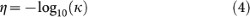with easy puzzles falling in the range 0 < η ≤ 1, medium ones in 1 < η ≤ 2, hard ones in 2 < η ≤ 3 and for ultra-hard puzzles η > 3.

We chose several instances from the “Sudoku of the Day” website20 in four of the categories defined there: easy (black square), medium (red circle), hard (green ×) and absurd (blue star). These ratings on the website try to estimate the hardness of puzzles when solved by humans. These ratings correlate very well with our hardness measure η, giving an average hardness value of 〈η〉 = 0.816 for easy, 〈η〉 = 1.439 medium, 〈η〉 = 1.782 for hard and 〈η〉 = 1.809 for what they call absurd. Another site we analyzed puzzles from is “Extreme Sudoku”25 (brown + signs on Fig.4). It claims to offer extremely hard Sudoku puzzles, their categories being: evil, excessive, egregious, excruciating and extreme. Indeed those puzzles are difficult with a range of η [1.1, 1.9] on the hardness scale, however, still far from the hardest puzzles we have found in the literature. Occasionally, daily newspapers present puzzles claimed to be the hardest Sudoku puzzles of the year. In particular, the escape rate for the Caveman Circus 2009 winner26 (turquoise diamond) and the Guardian 2010 hardest puzzle27 (maroon diamond) are indeed one order of magnitude smaller than the hardest puzzles on the daily Sudoku websites, placing them at η = 2.93 and η = 2.82 on the hardness scale. The USA Today 2006 hardest puzzle28, however, does not seem to be that hard for our algorithm having η = 2.17 (magenta diamond). Eppstein29 gives two Sudoku examples (orange left-pointing triangles) while describing his algorithm, one with η = 1.288 and a much harder one with η = 2.017. Elser et al.14 present an extremely hard Sudoku (black filled circle), which has an escape rate of κ = 0.0023 resulting in η = 2.639.

The smallest escape rates we have found are for the Sudokus listed as the hardest on Wikipedia22,23 (red triangles). The five puzzles, which we tested are called Platinum Blonde, Golden Nugget, Red Dwarf, coly013 and tarx0134. They have a hardness in the range 3 < η < 3.6, the ultra-hard Platinum Blonde (shown in Fig.2b) being the hardest with η = 3.5789 (κ = 0.00026).

While the escape rate correlates surprisingly well with human ratings of Sudoku hardness, it is natural to expect a correlation with the number of clues, d. Indeed, as a general rule of thumb, the fewer clues are given, the harder the puzzle, however, this is not universally true1. Here we tested a few instances with minimal30, that is 17 clues and almost minimal 18 clues (orange filled circles)31,32,33. As seen from Fig.4c, these are actually easier (1.2 < η < 2.4) than the hardest instances with more d = 21, 22 clues. In Fig.4d we then plot the escape rate as function of the constraint density α = M/N, leading to practically the same conclusion. This is because the constraint density α is essentially linearly correlated with the number of givens d, as shown in Fig.4e. The apparent non-monotonic behavior of puzzle hardness with the number of givens or constraint density is due to the fact that hardness cannot simply be characterized by a global, static variable such as d or α, but it also depends on the positioning pattern of the clues1.

## Discussion

Using the world of Sudoku puzzles, here we have presented further evidence that optimization hardness translates into complex dynamical behavior by an algorithm searching for solutions in an optimal fashion. Namely, there seems to be a trade-off between algorithmic performance and the complexity of the algorithm and/or its behavior. Simple, sequential search algorithms have a trivial description and simple dynamics, but an abysmal worst-case performance (2N), whereas algorithms that are among the best performers are complex in their description (instruction-list) and/or behavior (dynamics). This happens because in order to improve performance, algorithms have to exploit the structure of the problem one way or another. As hard problems have complex structures, the dynamics of the algorithms should be indicative of the problem's hardness. However, as a word of caution, observing complex dynamics performed by some black-box algorithm does not necessarily imply problem hardness. For example, one could consider any dynamical system that is guaranteed to visit all the 2N discrete states/configurations but perhaps with some arbitrary, complex behavior. This algorithm will always find solutions if they exist (by checking for satisfiability after every new state visited). But its instruction list would have no relevance to the problem itself (apart from the checking instructions to see if the new state satisfies the problem) and thus, it could take long times to find solutions even for problems that are otherwise easily solved by other algorithms. Hence, dynamical properties can only be regarded as descriptors of problem hardness if they are generated by algorithms that: 1) exploit the structure of the state space of the problem and 2) they show similar or better performance compared to other algorithms on the same problems.

The continuous-time dynamical system15 (2–3) as a deterministic algorithm does have these features: 1) the search happens on an energy landscapethat incorporates simultaneously all the constraints (problem structure) 2) it solves easy problems efficiently (polynomial time, both analog and discrete) and 3) it guarantees to find solutions to hard problems even for solvable cases where many other algorithms fail. Although it is not a polynomial cost algorithm, it seems to find solutions in continuous-time t that scales polynomially with N15. These features and the fact that the algorithm is formulated as a deterministic dynamical system with continuous variables, allows us to apply the theory of nonlinear dynamical systems on CTDS (2–3) to characterize the hardness of boolean satisfiability problems. In particular, via the measurable escape rate κ, or its negative log-value η, we can provide a single-scalar measure of hardness, well defined for any finite instance. We have illustrated this here on Sudoku puzzles, but the analysis can be repeated on any other ensemble from NP. Having a mathematically well-defined number to characterize optimization hardness for specific problems in NP provides more information than the polynomial/exponential-time solvability classification, or knowing what the constraint density α = M/N is (the latter being a non-dynamic/static measure). Moreover, within the framework of CTDS (2–3), dynamical systems and chaos theory methods can now be brought forth to help develop a novel understanding of optimization hardness.

## Methods

Type 1) constraints (main text) impose the uniqueness of the symbol Dij in a given cell, expressed as a +1-in-9-SAT constraint on the vector: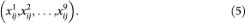that is, one and only one component in the boolean vector above can be TRUE (or 1), all others must be FALSE (or 0). Having 9 × 9 cells in the puzzle, this gives in total 81, +1-in-9-SAT constraints.

Type 2) constraints on rows, columns and sub-grids further impose that in every layer La we have the following 27, +1-in-9-SAT constraints: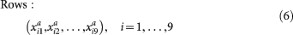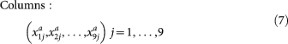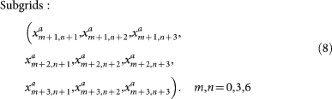with the same meaning as before, that is in all the boolean vectors above one and only one variable can be TRUE all others must be FALSE. Together with the 81 constraints of type 1) we thus have a total of 9 × 27 + 81 = 324 constraints in +1-in-9-SAT form.

Finally, type 3) constraints are imposed via d given digits or clues. It was only recently shown that uniqueness of a solution demands that d ≥ 1730. As discussed in the main text, each clue will eliminate 4 constraints: in its vertical tower, its column, its row and the 3×3 sub-grid containing the clue. For example, let us examine layer L4 (Fig.1c) of the puzzle shown in Fig.1a. There are three clues of 4 in cells (1, 9), (3, 3), (4, 4) and thus,,have to be fixed as TRUE in L4. In order to satisfy the constraints, the other variables in the same rows, columns, blocks and vertical columns must be set to FALSE. The unknown variables left in the SAT problem will be those in the light cells of Fig.1c. (The other clues will eliminate constraints and variables in other layers and vertical columns.) The total number of unknown variables N depends on d and on the placement of clues. The number of constraints is always 324 - 4d, however the number of variables in a clause can vary. For example in Fig.1c the constraint corresponding to the second row in layer L4 has only 2 unknown variables left (+1-in-2-SAT).

After the unknown boolean variables and the constraints have been identified we need to transform the formula into CNF. This is done easily, as any +1-in-k-SAT clause defined on the (y1, y2, …, yk) variables can be written as one k-SAT and k(k-1)/2 of 2-SAT constraints: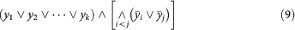The disjunction () of the first k variables enforces that at least one variable must be true, but the rest of (k(k - 1)/2) 2-SAT type constraints ensure that only one of them is allowed to be true.

## References

1. Rosenhouse, J. & Taalman, L. Taking Sudoku Seriously: The Math Behind the World's most Popular Pencil Puzzle (Oxford University Press, New York, 2011).

2. Garey, M. R. & Johnson, D. S. Computers and Intractability: A Guide to the Theory of NP-Completeness (W. H. Freeman & Co., New York, NY, USA, 1990).

3. Karp, R. M. Reducibility among combinatorial problems. In Complexity of Computer Computations., R.E. Miller and J.W. Thatcher (editors). Proc. of a Symp. on the Complexity of Computer Computations. (New York: Plenum. pp. 85–103, 1972).

4. Yato, T. & Seta, T. Complexity and completeness of finding another solution and its application to puzzles. IEICE Trans. Fundamentals E86-A(5), 1052–1060 (2003).

5. Fortnow, L. The status of the P versus NP problem. Commun. ACM 52, 78–86 (2009).

6. Barahona, F. On the computational complexity of Ising spin glass models. J. Phys. A: Math. Gen. 15, 3241–3253 (1982).

7. Istrail, S. Statistical Mechanics, Three-Dimensionality and NP-Completeness: I. Universality of Intractability of the Partition Functions of the Ising Model Across Non-Planar Lattices. Proceedings of the 32nd ACM Symposium on the Theory of Computing (STOC00), ACM Press, pp. 87–96 (2000)

8. Brueggemann, T. & Kern, W. An improved local search algorithm for 3-SAT. Theor. Comp. Sci. 329(1–3), 303313 (2004).

9. Ott, E. Chaos in Dynamical Systems 2nd edn (Cambridge Univ. Press, 2002).

10. Cencini, M., Cecconi, F. & Vulpiani, A. Chaos: from simple models to complex systems (World Scientific, Singapore, 2009).

11. Lai, Y.-C. & Tél, T. Transient Chaos: Complex Dynamics on Finite-Time Scales (Springer 2011).

12. Cvitanović, P., Artuso, R., Mainieri, R. Tanner, G. & Vattay, G. Chaos: Classical and Quantum, ChaosBook.org/version13 (Niels Bohr Institute, Copenhagen 2010).

13. Tél, T. & Gruiz, T. Chaotic dynamics: and introduction. (Cambridge University Press, 2006).

14. Elser, V., Rankenburg, I. & Thibault, P. Searching with iterated maps. Proc. Natl. Acad. Sci. USA 104, 418–423 (2007).

15. Ercsey-Ravasz, M. & Toroczkai, Z. Optimization hardness as transient chaos in an analog approach to constraint satisfaction. Nature Physics 7, 966–970 (2011).

16. Kadanoff, L. P. & Tang, C. Escape from strange repellers. Proc. Natl Acad. Sci. 81, 1276–1279 (1984).

17. Tél, T. & Lai, Y-C. Chaotic transients in spatially extended systems. Phys. Rep. 460, 245–275 (2008).

18. Zdeborová, L. & Mézard, M. Locked constraint satisfaction problems. Phys. Rev. Lett. 101, 078702 (2008).

19. Zdeborová, L. & Mézard, M. Constraint satisfaction problems with isolated solutions are hard. J. Stat. Mech.: Theor. Exp. P12004 (2008).

20. http://www.sudokuoftheday.co.uk (accessed on 26-07-2012)

23. http://en.wikipedia.org/wiki/Algorithmics_of_sudoku#Exceptionally_difficult_Sudokus_.28hard (accessed on 27-07-2012).

25. http://www.extremesudoku.info/sudoku.html (accessed on 26-07-2012).

26. http://cavemancircus.com/2009/11/05/the-hardest-sudoku-puzzle-ever/ (accessed on 26-07-2012).

27. Hutchinson, P. The world's hardest Sudoku? The Guardian, August 22 (2010) http://www.guardian.co.uk/media/2010/aug/22/worlds-hardest-sudoku (accessed on 21-07-2012).

28. http://www.usatoday.com/news/offbeat/2006-11-06-sudoku_x.htm (accessed on 26-07-2012).

29. Eppstein, D. Solving Single-digit Sudoku Subproblems, http://arxiv.org/abs/1202.5074v2

30. McGuire, G., Tugeman, B. & Civario, G. There is no 16-Clue Sudoku: Solving the Sudoku Minimum Number of Clues Problem. http://arxiv.org/abs/1201.0749 (accessed on 25-07-2012).

31. http://mapleta.maths.uwa.edu.au/gordon/sudokumin.php (accessed on 26-07-2012).

32. http://en.wikipedia.org/wiki/File:Symmetrical_18_clue_sudoku_01.JPG (accessed on 15-07-2012).

33. http://www.flickr.com/photos/npcomplete/3603730706/ (accessed on 24-06-2012).

## Acknowledgements

This work was supported in part by a grant of the Romanian National Authority for Scientific Research, CNCS-UEFISCDI, grant number PN-II-RU-TE-2011-3-0121 (MER) and by a University of Notre Dame internal capitalization grant (ZT).

## Author information

Authors

### Contributions

The authors contributed analysis and tools equally. Both authors wrote and reviewed the manuscript.

## Ethics declarations

### Competing interests

The authors declare no competing financial interests.

## Rights and permissions

Reprints and Permissions

Ercsey-Ravasz, M., Toroczkai, Z. The Chaos Within Sudoku. Sci Rep 2, 725 (2012). https://doi.org/10.1038/srep00725

• Accepted:

• Published: